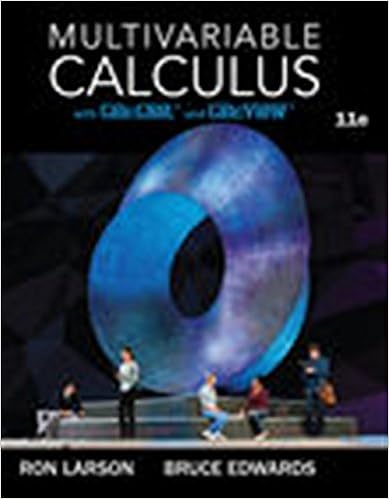# In the model should be large relative to mse formally

• Notes
• 38
• 92% (13) 12 out of 13 people found this document helpful

This preview shows page 35 - 38 out of 38 pages.

##### We have textbook solutions for you!
The document you are viewing contains questions related to this textbook.The document you are viewing contains questions related to this textbook.
Chapter 15 / Exercise 72
Multivariable Calculus
LarsonExpert Verified
in the model should be large (relative to MSE) Formally if all model assumption hold F = ( SSR ( ˆ β ) - SSR ( ˆ β A )) /p B MSE H 0 F ( p b , n - p - 1) If F > F α ( p B , n - p - 1), then we reject H 0 with significance level α , O.W., H 0 is not rejected. Note that SST - SSR ( ˆ β ) = SSE SST - SSR ( ˆ β A ) = SSE 0 where SSE 0 is sum of squares of residuals leaving out X B (fitting the model subject to H 0 ) Then the difference SSE 0 - SSE = SSR ( ˆ β ) - SSR ( ˆ β A ) Thus if the extra sum of squares of regression is small: 35
##### We have textbook solutions for you!
The document you are viewing contains questions related to this textbook.The document you are viewing contains questions related to this textbook.
Chapter 15 / Exercise 72
Multivariable Calculus
LarsonExpert Verified
The two models have similar residual sum of squares = the two models fit about the source we choose simpler model = we do not reject H 0 . Mathematically, F = ( SSR ( ˆ β ) - SSR ( ˆ β A )) /p B MSE = ( SSE 0 - SSE ) /p B MSE 8.2.2 The general linear hypothesis To test the very general hypothesis concerning the regression coefficients β H 0 : = b where T is a c × ( p + 1) matrix of constance, and b is a c × 1 vector of constance. For example, y = β 0 + β 1 x 1 + β 2 x 2 + β 3 x 3 + the null hypothesis H 0 : β 0 = 0 and β 1 = β 2 1 0 0 0 0 1 - 1 0 β 0 β 1 β 2 β 3 = 0 0 thus H 0 : = b . To test H 0 : = b in general 1. Fit regression with no constrains 2. Compute SSE 3. Fit regression model subject to constrains 4. Compute the new SSE 0 5. Compute F-ratio F = ( SSE 0 - SSE ) /c SSE/ ( n - p - 1) 6. If F > F α ( c, n - p - 1), then reject the null hypothesis; otherwise, not reject. Consider H 0 : β 2 = β 3 = 0 0 0 1 0 0 0 0 1 β 0 β 1 β 2 β 3 = 0 0 36
8.3 Categorical Predictors and InteractionTerms 8.3.1 Binary predictor Recall low both weight infant example: y : head circa x 1 : best age x 2 : toxaemia , 1 = “yes” , 0 = “No” Consider model y = β 0 + β 1 x 1 + β 2 x 2 + ˆ y = 1 . 496 + 0 . 874 x 1 - 1 . 412 x 2 + testing β 2 = 0 It is often not reasonable to assume the effect of other explanatory variables are some across different groups Interaction terms: y = β 0 + β 1 x 1 + β 2 x 2 + β 3 x 1 x 2 + = ( y = β 0 + β 1 x 1 + if x 2 = 0 y = β 0 + β 2 + ( β 1 + β 3 ) x 1 + if x 2 = 1 by adding interaction term, it allows X 1 to have a different effect on y depending on the value of x 2 . 8.3.2 Hypothesis Testing of Interaction Term H 0 : β 3 = 0 v.s. H a : β 3 6 = 0 tells whether the effect is different or not between groups. 8.3.3 categorical predictor with more than 2 levels Example y : prestige score of occupations exp. var : education (in years) , income type of occupation : blue collar, white collar, professional Dummy variable : D 1 = ( 1 professional 0 O. W. , D 2 = ( 1 white collar 0 O. W. 37
type of occupation D 1 D 2 professional 1 0 white collar 0 1 blue collar 0 0 The categorical exp var. with k levels can be represented by k - 1 dummies. The regression model y = β 0 + β 1 x 1 + β 2 x 2 + β 3 D 1 + β 4 D 2 + prof y = ( β 0 + β 3 ) + β 1 x 1 + β 2 x 2 + w.c. y = ( β 0 + β 4 ) + β 1 x 1 + β 2 x 2 + b.c. y = β 0 + β 1 x 1 + β 2 x 2 + where β 3 represents the constant vertical distance between the paralleled regression planes for prof and b.c. occupation. β 4 represents the constant vertical distance between the paralleled regression planes for w.c. and b.c. occupation.
•••